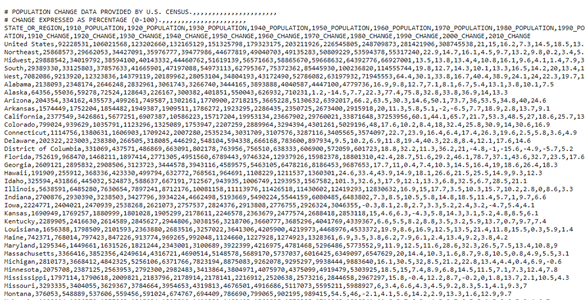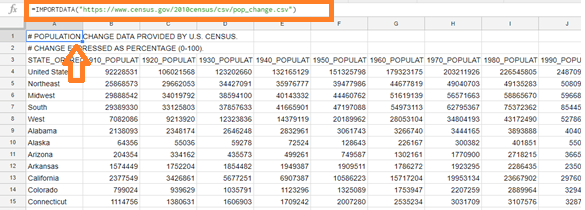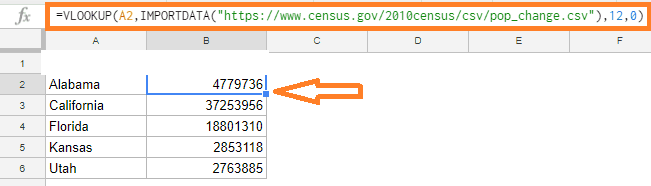# How to Use IMPORTDATA function in Google Sheets? (Fetch CSV/TSV File)

We can use the IMPORTDATA functions to import a Comma-Separated Value (.CSV) file and/or a Tab Separated Value (.TSV) file into Google Sheets. This means that If you wish to import data from a.CSV or.TSV file from the web into Google Sheets, the IMPORTDATA function comes in helpful. When dealing with tabular data such as sales, population, and statistics, it is often employed. All we have to do is to affix the URL along with the IMPORTDATA formula to import the data.

## IMPORTDATA Function Syntax

Before understanding how to import the data with the help of IMPORTDATA function, let’s understand how to use the IMPORTADATA function in Google Sheets.

=IMPORTDATA(“URL”)

There is only one argument to this function. You must include the entire URL of the file location in double quotes. You can specify the cell reference instead of the URL if the URL is in a cell in Google Sheets.

### How to Import Data using IMPORTDATA Function in Google Sheets?

Consider the following data. The URL of the data is- https://www.census.gov/2010census/csv/pop_change.csv. (Source: support.google.com). If you enter this data in the search engine, the following data will be displayed.It’s quite hard to understand this data since it’s drafted in .csv format. In order to understand the data and make the working easy, let’s import the data into Google Sheets. The steps this CSV data into Google Sheet are explained below:

• Step 2: Move the first cell.
• Step 3: Now enter the formula =IMPORTDATA(“https://www.census.gov/2010census/csv/pop_change.csv”).
• Step 4: Click on the “Enter” button.
• Step 5: You will see the data being imported into the Google Sheet as shown below.### How to Import Specified Data using IMPORTDATA Function?

In some instances, we might not require the entire data to be imported. Thus to import specified data from the CSV or TSV file into Google Sheets, we can simply modify the IMPORTDATA function with the help of the VLOOKUP function.

We can use the same URL that we used earlier and let’s start fetching the specified range of cells with the help of steps given below:

• Step 2: Move the cell where you would like to import the data.
• Step 3: Now enter the formula “=VLOOKUP(A2,IMPORTDATA(“https://www.census.gov/2010census/csv/pop_change.csv”),12)“.
• Step 4: Press the “Enter” button and you will see the results as shown below.Note that we utilized the IMPORTDATA function as the table array for the VLOOKUP function in the above formula. We picked 12 as the column number because we only needed statistics for the 2010 population (the third argument of the VLOOKUP function).

Things to Note

When utilizing the IMPORTDATA function in Google Sheets, there are a few things you should keep in mind:

• Based on the data in the CSV or tsv file, the function automatically fills the cells in the spreadsheet.
• The IMPORTDATA method would throw an error if some of the cells already had data in them. As a result, make sure you have enough empty cells to hold the data from the CSV or TSV files.
• Make sure you are using the correct URL version. It will give you an error if you use ‘HTTP instead of ‘HTTPS.’